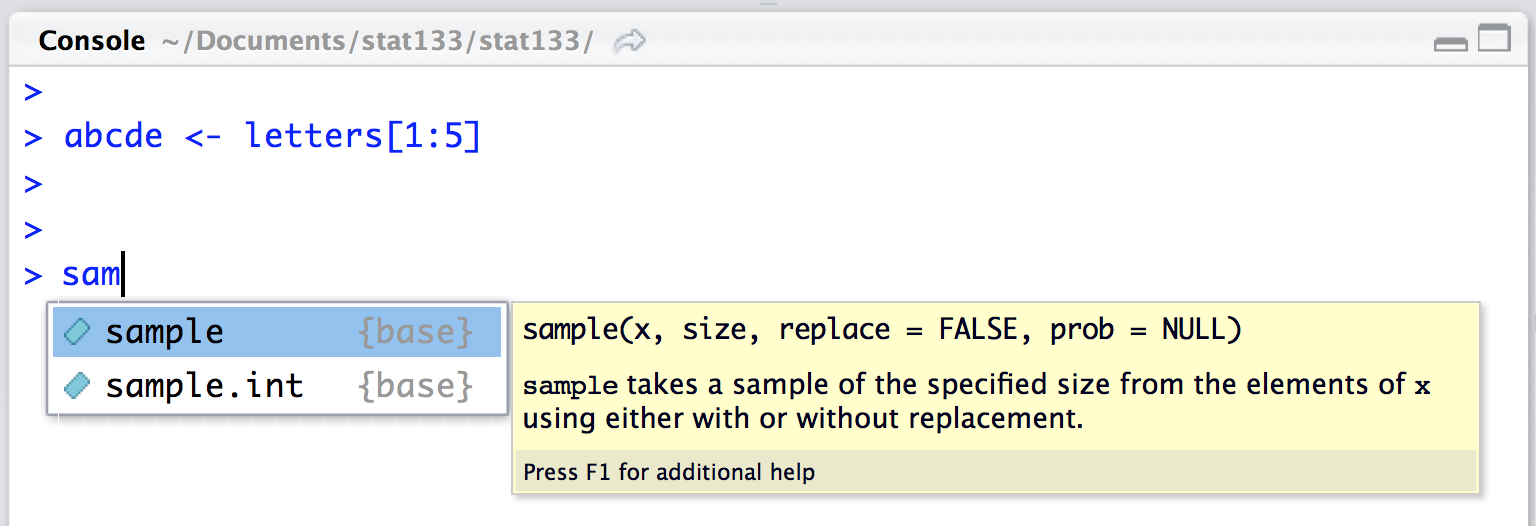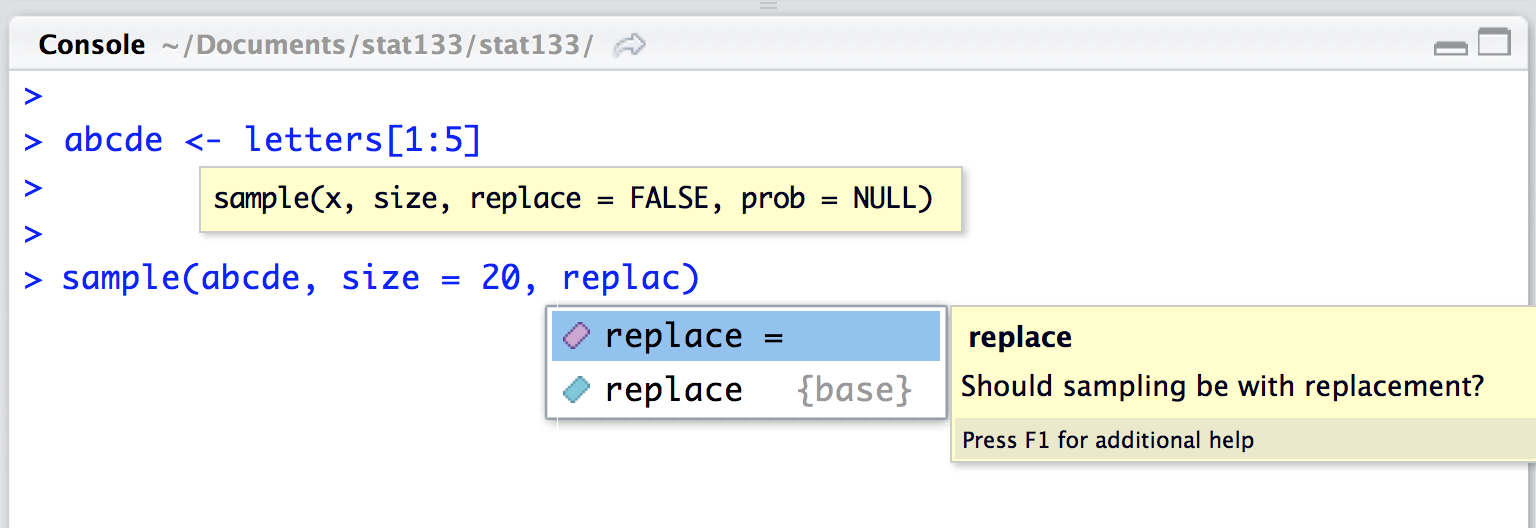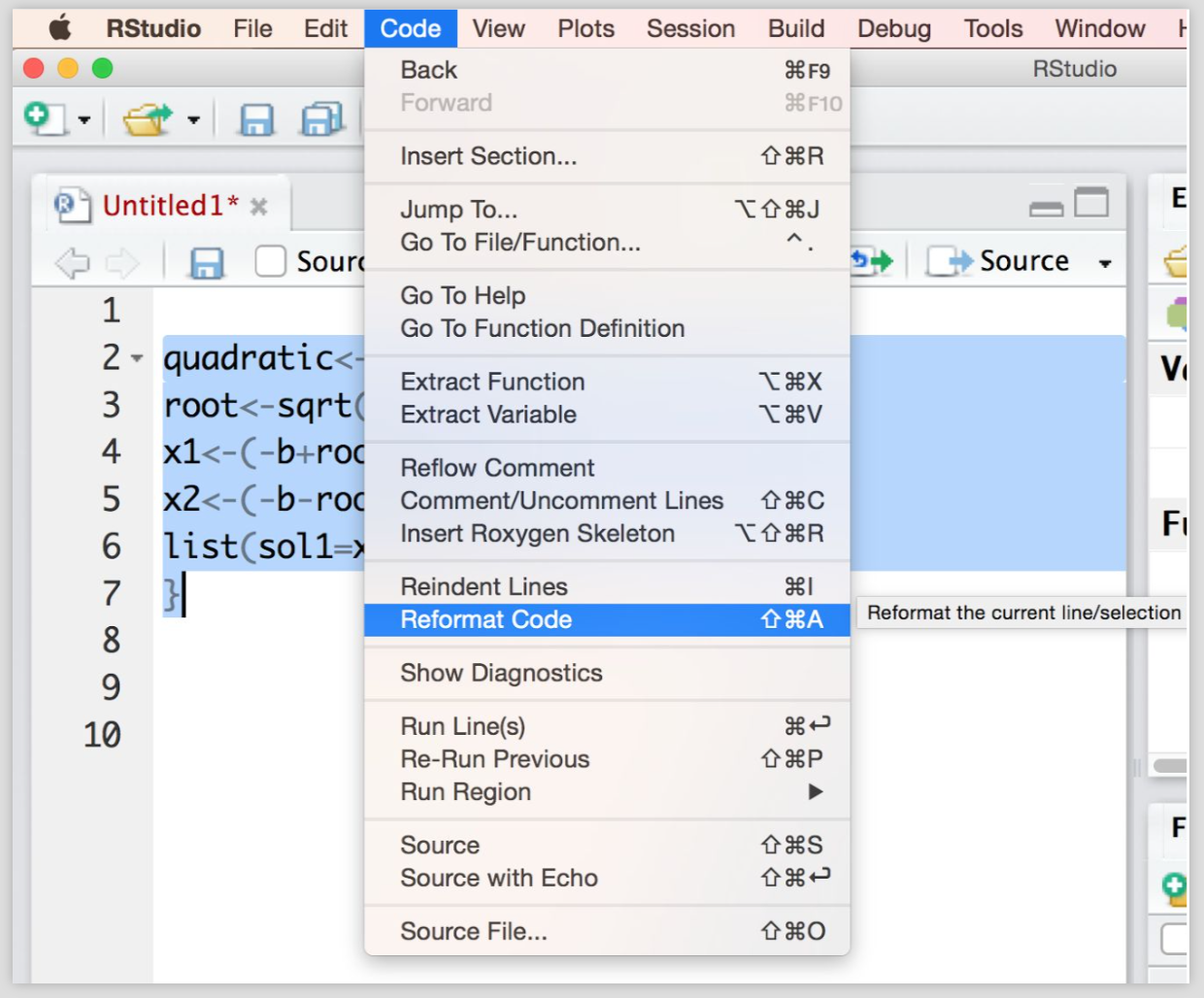28 Good Coding Practices

Now that you’ve worked with various R scripts, written some functions, and done some data manipulation, it’s time to look at some good coding practices.

Popular style guides among useR’s

28.1 Syntax Highlighting

Nowadays most text editors and IDE (e.g. RStudio) come with syntax highlighting features which make writing and reading code easier. However, it is still possible to find yourself in a situation where the editor you are using has no syntax highlighting. Let’s quickly compare the difference between a few lines of code with and without syntax highlighting:

without:

# without syntax highlighting
a <- 2
x <- 3
y <- log(sqrt(x))
3*x^7 - pi * x / (y - a)
"some strings"

versus with:

a <- 2
x <- 3
y <- log(sqrt(x))
3*x^7 - pi * x / (y - a)
"some strings"

Without highlighting it’s harder to detect syntax errors:

numbers <- c("one", "two, "three")

if (x > 0) {
3 * x + 19
} esle {
2 * x - 20
}

With highlighting it’s easier to detect syntax errors:

numbers <- c("one", "two, "three")

if (x > 0) {
3 * x + 19
} esle {
2 * x - 20
}

RStudio IDE has features of all good IDEs:

• Syntax highlighting
• Syntax aware
• Able to evaluate R codei
• by line
• by selection
• entire file
• Command completion

Use an IDE with autocompletionFigure 28.1: IDE with autocompletion

Use an IDE that provides helpful documentationFigure 28.2: IDE with help documentation

28.2 Good Source Code

Think about programs/scripts/code as works of literature (Literate Programming). Well readable by humans, and as much self-explaining as possible

“Let us change our traditional attitude to the construction of programs: Instead of imagining that our main task is to instruct a computer what to do, let us concentrate rather on explaining to human beings what we want a computer to do”. Donald Knuth (Literate Programming, 1984)

Literate programming recommendations:

• Choose the names of variables carefully
• Explain what each variable means
• Strive for a program that is comprehensible
• Introduce concepts in an order that is best for human understanding

Here’s an example of instructing a computer what to do

# good for computers (not much for humans)
if (is.numeric(x) & x > 0 & x %% 1 == 0) TRUE else FALSE

Can you guess what the above code is doing? It’s better to write code in a way that we explain a human being what we want a computer to do

# good for humans
is_positive_integer(x)

Better to write a function that is human-understandable, not just machine-understandable

# example
is_positive_integer <- function(x) {
(is.numeric(x) & x > 0 & x %% 1 == 0)
}

is_positive_integer(2)

is_positive_integer(2.1)

28.2.1 Indentation

• Keep your indentation style consistent
• There is more than one way of indenting code
• There is no “best” style that everyone should be following
• You can indent using spaces or tabs (but don’t mix them)
• Can help in detecting errors in your code because it can expose lack of symmetry
• Do this systematically (RStudio editor helps a lot)

Don’t write code like this:

# Don't do this!
if(!is.vector(x)) {
stop('x must be a vector')
} else {
if(any(is.na(x))){
x <- x[!is.na(x)]
}
total <- length(x)
x_sum <- 0
for (i in seq_along(x)) {
x_sum <- x_sum + x[i]
}
x_sum / total
}

# better with indentation
if(!is.vector(x)) {
stop('x must be a vector')
} else {
if(any(is.na(x))) {
x <- x[!is.na(x)]
}
total <- length(x)
x_sum <- 0
for (i in seq_along(x)) {
x_sum <- x_sum + x[i]
}
x_sum / total
}

There are several Indenting Styles

# style 1
find_roots <- function(a = 1, b = 1, c = 0)
{
if (b^2 - 4*a*c < 0)
{
return("No real roots")
} else
{
return(quadratic(a = a, b = b, c = c))
}
}

# style 2
find_roots <- function(a = 1, b = 1, c = 0) {
if (b^2 - 4*a*c < 0) {
return("No real roots")
} else {
return(quadratic(a = a, b = b, c = c))
}
}

Benefits of code indentation:

• Easier to understand
• Easier to modify
• Easier to maintain
• Easier to enhance

28.2.2 Reformat Code in RStudio

• RStudio provides code reformatting (use it!)
• Click Code on the menu bar
• Then click Reformat CodeFigure 28.3: Reformat code in RStudio

# unformatted code
root<-sqrt(b^2-4*a*c)
x1<-(-b+root)/2*a
x2<-(-b-root)/2*a
list(sol1=x1,sol2=x2)
}

# reformatted code
quadratic <- function(a = 1, b = 1, c = 0) {
root <- sqrt(b ^ 2 - 4 * a * c)
x1 <- (-b + root) / 2 * a
x2 <- (-b - root) / 2 * a
list(sol1 = x1,sol2 = x2)
}

28.2.3 Meaningful Names

Choose a consistent naming style for objects and functions

• someObject (lowerCamelCase)
• SomeObject (UpperCamelCase)
• some_object (underscore separation)
• some.object (dot separation)

Avoid using names of standard R objects, for example:

• vector
• mean
• list
• data
• c
• colors

If you’re thinking about using names of R objects, prefer something like this

• xvector
• xmean
• xlist
• xdata
• xc
• xcolors

Better to add meaning like this

• mean_salary
• input_vector
• data_list
• data_table
• first_last
• some_colors

Here’s a quiz example, what does the following functino getThem() do?

getThem <- function(values, y) {
list1 <- c()

for (i in values) {
if (values[i] == y)
list1 <- c(list1, x)
}
return(list1)
}

this is more meaningful:

getFlaggedCells <- function(gameBoard, flagged) {
flaggedCells <- c()

for (cell in gameBoard) {
if (gameBoard[cell] == flagged)
flaggedCells <- c(flaggedCells, x)
}
return(flaggedCells)
}

Also, better to use meaningful distinctions

# argument names 'a1' and 'a2'?
move_strings <- function(a1, a2) {
for (i in seq_along(a1)) {
a1[i] <- toupper(substr(a1, 1, 3))
}
a2
}

# argument names
move_strings <- function(origin, destination) {
for (i in seq_along(origin)) {
destination[i] <- toupper(substr(origin, 1, 3))
}
destination
}

Prefer Pronounceable Names

# cryptic abbreviations
DtaRcrd102 <- list(
nm = 'John Doe',
bdg = 'Valley Life Sciences Building',
rm = 2060
)

# pronounceable names
Customer <- list(
name = 'John Doe',
building = 'Valley Life Sciences Building',
room = 2060
)

28.2.4 White Spaces

• Use a lot of it
• around operators (assignment and arithmetic)
• between function arguments and list elements
• between matrix/array indices, in particular for missing indices
• Split long lines at meaningful places

Avoid this

a<-2
x<-3
y<-log(sqrt(x))
3*x^7-pi*x/(y-a)

Much Better

a <- 2
x <- 3
y <- log(sqrt(x))
3*x^7 - pi * x / (y - a)

Another example:

# Avoid this
plot(x,y,col=rgb(0.5,0.7,0.4),pch='+',cex=5)

# okay
plot(x, y, col = rgb(0.5, 0.7, 0.4), pch = '+', cex = 5)

Another readability recommendation is to limit the width of line: they should be broken/wrapped around so that they are less than 80 columns wide

# lines too long
histogram <- function(data){
hist(data, col = 'gray90', xlab = 'x', ylab = 'Frequency', main = 'Histogram of x')
abline(v = c(min(data), max(data), median(data), mean(data)),
col = c('gray30', 'gray30', 'orange', 'tomato'), lty = c(2,2,1,1), lwd = 3)
}

Lines should be broken/wrapped aroung so that they are less than 80 columns wide

# lines with okay width
histogram <- function(data) {
hist(data, col = 'gray90', xlab = 'x', ylab = 'Frequency',
main = 'Histogram of x')
abline(v = c(min(data), max(data), median(data), mean(data)),
col = c('gray30', 'gray30', 'orange', 'tomato'),
lty = c(2,2,1,1), lwd = 3)
}

28.2.5 White spaces

• Spacing forms the second important part in code indentation and formatting.
• Spacing makes the code more readable
• Use spacing consistently
# this can be improved
stats <- c(min(x), max(x), max(x)-min(x),
quantile(x, probs=0.25), quantile(x, probs=0.75), IQR(x),
median(x), mean(x), sd(x)
)

Don’t be afraid of splitting one long line into individual pieces:

# much better
stats <- c(
min(x),
max(x),
max(x) - min(x),
quantile(x, probs = 0.25),
quantile(x, probs = 0.75),
IQR(x),
median(x),
mean(x),
sd(x)
)

You can even do this:

# also OK
stats <- c(
min    = min(x),
max    = max(x),
range  = max(x) - min(x),
q1     = quantile(x, probs = 0.25),
q3     = quantile(x, probs = 0.75),
iqr    = IQR(x),
median = median(x),
mean   = mean(x),
stdev  = sd(x)
)
• All commas and semicolons must be followed by single whitespace
• All binary operators should maintain a space on either side of the operator
• Left parenthesis should start immediately after a function name
• All keywords like if, while, for, repeat should be followed by a single space.

All binary operators should maintain a space on either side of the operator

# NOT Recommended
a=b-c
a = b-c
a=b - c;

# Recommended
a = b - c

All binary operators should maintain a space on either side of the operator

# Not really recommended
z <- 6*x + 9*y

# Recommended (option 1)
z <- 6 * x + 9 * y

# Recommended (option 2)
z <- (7 * x) + (9 * y)

Left parenthesis should start immediately after a function name

# NOT Recommended

# Recommended

All keywords like if, while, for, repeat should be followed by a single space.

if(is.numeric(object)) {
mean(object)
}

# much better
if (is.numeric(object)) {
mean(object)
}

28.2.6 Syntax: Parentheses

Use parentheses for clarity even if not needed for order of operations.

a <- 2
x <- 3
y <- 4

a/y*x

# better
(a / y) * x

another example

# confusing
1:3^2
#>  1 2 3 4 5 6 7 8 9

# better
1:(3^2)
#>  1 2 3 4 5 6 7 8 9

• But don’t belabor the obvious
• Use blank lines to separate blocks of code and comments to say what the block does
• Remember that in a few months, you may not follow your own code any better than a stranger
• Some key things to document:
• summarizing a block of code
• explaining a very complicated piece of code
• explaining arbitrary constant values

MV <- get_manifests(Data, blocks)
check_MV <- test_manifest_scaling(MV, specs$scaling) gens <- get_generals(MV, path_matrix) names(blocks) <- gens$lvs_names
block_sizes <- lengths(blocks)
blockinds <- indexify(blocks)

# ==================================================
# Preparing data and blocks indexification
# ==================================================
# building data matrix 'MV'
MV <- get_manifests(Data, blocks)
check_MV <- test_manifest_scaling(MV, specs$scaling) # generals about obs, mvs, lvs gens <- get_generals(MV, path_matrix) # indexing blocks names(blocks) <- gens$lvs_names
block_sizes <- lengths(blocks)
blockinds <- indexify(blocks)

Different line styles:

####################################################

# ==================================================

# **************************************************

# --------------------------------------------------

for example:

# ==================================================
# Preparing data and blocks indexification
# ==================================================
# building data matrix 'MV'
MV <- get_manifests(Data, blocks)
check_MV <- test_manifest_scaling(MV, specs$scaling) or this one # ---- Preparing data and blocks indexification ---- # building data matrix 'MV' MV <- get_manifests(Data, blocks) check_MV <- test_manifest_scaling(MV, specs$scaling)

Include comments to say what a block does, or what a block is intended for

# =====================================================
# Data: liga2015
# =====================================================
# For this session we'll be using the dataset that
# comes in the file 'liga2015.csv' (see github repo)
# This dataset contains basic statistics from the
# Spanish soccer league during the season 2014-2015

Another example

x <- matrix(1:10, nrow = 2, ncol = 5)

# mean vectors by rows and columns
xmean1 <- apply(x, 1, mean)
xmean2 <- apply(x, 2, mean)

# Subtract off the mean of each row/column
y <- sweep(x, 1, xmean1)
z <- sweep(x, 2, xmean2)

# Multiply by the mean of each column (for some reason)
w <- sweep(x, 2, xmean1, FUN = "*")

Be careful with your comments (you never know who will end up looking at your code, or where you’ll be in the future)

# F***ing piece of code that drives me bananas

# wtf function

# best for loop ever

28.2.8 Source Code Files

• Break code into separate files (<2000-3000 lines per file)
• Give files meaningful names
• Group related functions within a file

• Who wrote / programmed it
• When was it done
• What is it all about
• How the code might fit within a larger program

# ===================================================
# Some Title
# Author(s): First Last
# Date: month-day-year
# Description: what this code is about
# Data: perhaps is designed for a specific data set
# ===================================================

If you need to load R packages, do so at the beginning of your script, after the header:

# ===================================================
# Some Title
# Author(s): First Last
# Date: month-day-year
# Description: what this code is about
# Data: perhaps is designed for a specific data set
# ===================================================

library(stringr)
library(ggplot2)
library(MASS)

28.3 Don’t Repeat Yourself

The famour DRY principle

Every piece of knowledge must have a single, unambiguous, authoritative representation within a system.

Many people write code like this:

# avoid repetition
plot(x, y, type = 'n')
points(x[size == 'xsmall'], y[size == 'xsmall'], col = 'purple')
points(x[size == 'small'], y[size == 'small'], col = 'blue')
points(x[size == 'medium'], y[size == 'medium'], col = 'green')
points(x[size == 'large'], y[size == 'large'], col = 'orange')
points(x[size == 'xlarge'], y[size == 'xlarge'], col = 'red')

There’s a lot of repetition in the previous code chunk; this can be solved with the use of a for() loop:

# avoid repetition
size_colors <- c('purple', 'blue', 'green', 'orange', 'red')
plot(x, y, type = 'n')
for (i in seq_along(levels(size))) {
points(x[size == i], y[size == i], col = size_colors[i])
}

28.3.1 Look at other people’s code

Look at other people’s code

• It takes time to develop a personal style
• Try different styles and see which one best fits you
• Sometimes you have to adapt to a company’s style
• There is no one single best style

28.3.2 Exercises

What’s wrong with this function?

average <- function(x) {
l <- length(x)
for(i in l) {
y[i] <- x[i]/l
z <- sum(y[1:l])
return(as.numeric(z))
}
}

What’s wrong with this function?

freq_table <- function(x) {
table <- table(x)
'category' <- levels(x)
'count' <- print(table)
'prop' <- table/length(x)
'cumcount' <- print(table)
'cumprop' <- table/length(x)
if(is.factor(x)) {
return(data.frame(rownames=c('category', 'count','prop',
'cumcount','cumprop')))
} else {
stop('Not a factor')
}
}
• What other suggestions do you have?
• How could we restructure the code, to make it easier to read?
• Grab a buddy and practice “code review”. We do it for methods and papers, why not code?
• Our code is a major scientific product and the result of a lot of hard work!